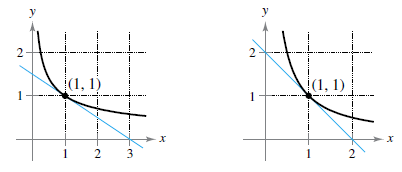Chapter 2.2, Problem 6E

Chapter
Section
Textbook Problem

Estimating Slope In Exercises 5 and 6, use the graph to estimate the slope of the tangent line to y = x n at the point (1.1). Verify your answer analytically. To print an enlarged copy of the graph, go to Math Graph. com.(a) y = x − 1 / 2 (b) y = x − 1(a)

To determine

To calculate: The slope of the tangent line to y=x1/2 at the point (1,1) by using the provided graph and verify the answer analytically.

Explanation

Given:

The function is: y=x1/2 which has the graph provided below,

Formula used:

The slope of the line passing through the points (x1,y1) and (x2,y2), is given by,

m=y2y1x2x1

The slope of a graph of a function is the derivative of that function, as below,

m=ddx[f(x)]

The power rule of differentiation states that,

ddx[xn]=nxn1

Calculation:

Consider the graph,

Observe that the tangent line crosses the y-axis at y=32.

So, the y-intercept of the tangent line is (0,32).

The next point is (1,1).

So, the slope of the line passes through the point (0,32) and (1,1) is,

m=13210=12

Therefore, the slope of the tangent line is 12

(b)

To determine

To calculate: The slope of the tangent line to y=x1 at the point (1,1) by using the provided graph and verify the answer analytically.

Still sussing out bartleby?

Check out a sample textbook solution.

See a sample solution

The Solution to Your Study Problems

Bartleby provides explanations to thousands of textbook problems written by our experts, many with advanced degrees!

Get Started

Evaluate the expression and write your answer in the form a + bi. 10. 343i

Single Variable Calculus: Early Transcendentals, Volume I

16. Compute .

Mathematical Applications for the Management, Life, and Social Sciences

For y = x3 ln x, y = a) x2(1 + 3 ln x) b) x3 + 3x2 (ln x) c) 3x d) 4x2(ln x)

Study Guide for Stewart's Single Variable Calculus: Early Transcendentals, 8th

For a = 2i + 3j − 4k and b = −i + 2j − k, a · b = 0 8 10 12

Study Guide for Stewart's Multivariable Calculus, 8th

List the major sections of an APA-style report in order of appearance.

Research Methods for the Behavioral Sciences (MindTap Course List)

Evaluate each expression. (55)(66)(77)(88)

College Algebra (MindTap Course List)